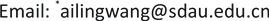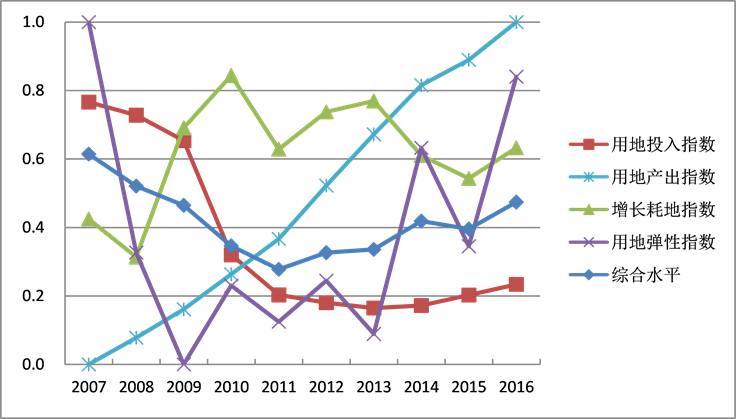﻿ 基于熵权法和TOPSIS模型的龙口市建设用地集约利用评价 Evaluation of Construction Land Intensive Use Based on Entropy Weight Method and TOPSIS Model of Longkou City

Urbanization and Land Use
Vol.06 No.02(2018), Article ID:25442,8 pages
10.12677/ULU.2018.62005

Evaluation of Construction Land Intensive Use Based on Entropy Weight Method and TOPSIS Model of Longkou City

Chenchen Su, Ao Zhou, Xi Liu, Ailing Wang*

College Resources and Environment, Shandong Agricultural University, Tai’an ShandongReceived: May 24th, 2018; accepted: Jun. 8th, 2018; published: Jun. 15th, 2018ABSTRACT

The evaluation of construction land intensive use is of great significance to tapping the potential of construction land and improving the level of construction land intensive use. Taking Longkou City as the research area, this paper selected 12 indicators to establish the evaluation index system, which included land input, land output, land increasing consumption and land use elasticity aspects, and by the aid of entropy weight method and TOPSIS model evaluated the status and changes of construction land intensive use from 2007 to 2016. The results showed that the input level of construction land experienced process from declining to rising, the output level of construction land showed an overall growth trend, the level of land increasing consumption experienced process from declining to fluctuant rising, the level of land use elasticity experienced process from rapidly declining to fluctuant rising, and the comprehensive level of construction land intensive use of Longkou city had a downside from 2007 to 2011, and showed a slowly rising trend from 2012 to 2016. Lastly, some suggestions were given about construction land intensive use according to the researching results, such as strictly controlling the total scale of construction land, strengthening the management of rural idle homestead and carrying out remediation of village land to improve the intensity of village land use.

Keywords:Construction Land, Intensive Use Evaluation, Entropy Weight Method, TOPSIS Model, Longkou City1. 引言

2. 研究区概况和数据来源

2.1. 研究区概况

2.2. 数据来源

3. 研究方法

TOPSIS (Technique for Order Preference by Similarity to an Ideal Solution)模型称为“逼近理想解排序方法”，是根据评价对象与理想化目标的接近程度进行排序的方法，是一种距离综合评价方  。具体思路为通过假定正理想解与负理想解，测算各评价对象与正、负理想解的距离而得到其与理想方案的相对贴近度，进行各评价对象的优劣排序   。该方法能够客观反映评价对象的优劣。具体步骤如下：

1) 构建加权矩阵。将标准化矩阵Pij与权重向量wj相乘得到加权后的规范化矩阵V，如式(1)。

$V={\left({v}_{ij}\right)}_{m×n}=\left({p}_{ij}×{w}_{j}\right)$ (1)

2) 确定正理想解和负理想解。从加权矩阵中找出各指标的最大值构成正理想解V+，最小值构成负理想解V，如式(2)。

$\begin{array}{l}{V}^{+}=\left\{\mathrm{max}{v}_{ij}|i=1,2,\cdots ,m\right\}=\left({v}_{1}{}^{+},{v}_{2}{}^{+},\cdots ,{v}_{m}^{+}\right)\\ {V}^{-}=\left\{\mathrm{min}{v}_{ij}|i=1,2,\cdots ,m\right\}=\left({v}_{1}^{-},{v}_{2}^{-},\cdots ,{v}_{m}^{-}\right)\end{array}$ (2)

3) 距离计算。计算各评价对象值与正理想解的距离D+和负理想解的距离D，如式(3)

$\begin{array}{l}{D}_{i}^{+}=\sqrt{\sum _{i=1}^{m}{\left({v}_{ij}-{v}_{i}^{+}\right)}^{2}}\text{\hspace{0.17em}}\text{\hspace{0.17em}}\left(i=1,2,\cdots ,m\right)\\ {D}_{i}^{-}=\sqrt{\sum _{i=1}^{m}{\left({v}_{ij}-{v}_{i}^{-}\right)}^{2}}\text{\hspace{0.17em}}\text{\hspace{0.17em}}\left(i=1,2,\cdots ,m\right)\end{array}$ (3)

4) 计算各评价对象与最优方案的贴近度Ci，如式(4)

${C}_{i}=\frac{{D}_{i}^{-}}{{D}_{i}^{+}+{D}_{i}^{-}}$ (4)

4. 龙口市建设用地集约利用评价

4.1. 熵权法

1) 假设评价年份有m个，评价指标有n个，建立原始数据矩阵X，如式(5)。

$X={\left({x}_{ij}\right)}_{m×n}\left(i=1,2,\cdots ,m,j=1,2,\cdots ,n\right)$ (5)

2) 对原始指标进行标准化处理，如式(6)。

${{X}^{\prime }}_{ij}=\frac{{x}_{ij}-\mathrm{min}{x}_{j}}{\mathrm{max}{x}_{j}-\mathrm{min}{x}_{j}}$

${{X}^{\prime }}_{ij}=\frac{\mathrm{max}{x}_{j}-{x}_{ij}}{\mathrm{max}{x}_{j}-\mathrm{min}{x}_{j}}$ (6)

3) 计算信息熵ej

${e}_{j}=-k\sum _{i=1}^{m}{p}_{ij}\mathrm{ln}\left({p}_{ij}\right)$ (7)

4) 计算指标的权重wj

${w}_{j}=\frac{1-{e}_{j}}{\sum _{j=1}^{n}1-{e}_{j}}$ (8)

4.2. 指标体系构建

4.3. 指标权重确定

4.4. 准则层评价Table 1. The evaluation index system of construction land intensive useTable 2. The weights of construction land intensive use evaluation indexFigure 1. Indictors change of construction land intensive use

4.5. 综合评价

5. 结果分析

5.1. 用地投入指数

5.2. 用地产出指数

5.3. 增长耗地指数

5.4. 用地弹性指数

5.5. 综合指数

2007~2016年间龙口市建设用地集约利用综合水平整体呈缓慢下降–缓慢上升趋势。2007~2011年，缓慢下降阶段，集约利用水平逐渐下降，2011年达到最低值0.2779，2007年贴近度分值是2011年的2.21倍。2012~2016年，缓慢上升阶段，2012年开始，龙口市建设用地集约利用水平呈现缓慢增长趋势，2012年贴近度分值为0.3263，到2016年提高至0.4738，年均增长率为9.045%。

6. 结论与建议

6.1. 结论

1) 参考建设用地集约评价规程及相关文献，考虑龙口市特点，从投入产出、用地强度、增长耗地、用地弹性4方面选取12个指标，构建包括指标层、准则层的龙口市建设用地集约利用评价指标体系，并采用熵权法确定指标权重。

2) 2007~2016年间，龙口市建设用地投入水平指数呈“下降–上升”趋势，用地产出指数持续提高，增长耗地指数整体呈“下降–波动上升”态势，用地弹性指数呈现“快速下降–波动上升”趋势，综合指数整体呈“缓慢下降–缓慢上升”趋势。

6.2. 建议

Evaluation of Construction Land Intensive Use Based on Entropy Weight Method and TOPSIS Model of Longkou City[J]. 城镇化与集约用地, 2018, 06(02): 30-37. https://doi.org/10.12677/ULU.2018.62005

1. 1. 曹银贵, 袁春, 周伟, 王静, 钱铭杰. 基于主成分分析的全国建设用地集约度评价[J]. 生态环境, 2008, 17(4): 1657-1661.

2. 2. 石培基, 邴广路. 基于熵值法的建设用地集约利用评价——以甘肃省为例[J]. 干旱区研究, 2009, 26(4): 502-507.

3. 3. 曲衍波, 张勇, 李慧燕, 高宇. 基于“五量”协同模型的山东省建设用地集约利用评价及障碍调控[J]. 资源科学, 2017, 39(6): 1013-1025.

4. 4. 张俊平, 胡月明, 田原, 王璐, 刘素萍. 广东省县级建设用地集约利用综合评价——以紫金县为例[J]. 应用生态学报, 2010, 21(2): 422-428.

5. 5. 曹银贵, 郑新奇, 胡业翠. 区域建设用地集约利用评价研究——以济南市为例[J]. 经济地理, 2010, 30(6): 1016-1020.

6. 6. 中华人民共和国国土资源部. TD/T 1018-2008. 建设用地节约集约利用评价规程[S]. 北京: 中华人民共和国国土资源部, 2008.

7. 7. 程佳, 孔祥斌, 赵晶, 张雪靓. 基于主体功能区的大都市区域建设用地集约利用评价——以北京市为例[J]. 中国农业大学学报, 2013, 18(6): 207-215.

8. 8. 信桂新, 杨朝现, 杨庆媛, 李承桧, 魏朝富. 用熵权法和改进TOPSIS模型评价高标准基本农田建设后效应[J]. 农业工程学报, 2017, 33(1): 238-249.

9. 9. 李灿, 张凤荣, 朱泰峰, 奉婷, 安萍莉. 基于熵权TOPSIS模型的土地利用绩效评价及关联分析[J]. 农业工程学报, 2013, 29(5): 217-227.

10. 10. 庄伟, 廖和平, 潘卓, 杨伟, 李涛, 张甜. 基于变权TOPSIS模型的三峡库区土地生态安全评估——以巫山县为例[J]. 西南大学学报(自然科学版), 2014, 36(8): 106-112.

11. 11. 余华银, 李超, 黄萍. 熵值法在EXCEL中的VBA实现[J]. 统计教育, 2004(3): 12-14.

12. 12. 雷勋平, Robin Qiu, 刘勇. 基于熵权TOPSIS模型的区域土地利用绩效评价及障碍因子诊断[J]. 农业工程学报, 2016, 32(13): 243-253.

13. 13. 杜挺, 谢贤健, 梁海艳, 黄安, 韩全芳. 基于熵权TOPSIS和GIS的重庆市县域经济综合评价及空间分析[J]. 经济地理, 2014, 34(6): 40-47.

14. 14. 智刚, 李秀霞, 孙占海. 中国城市建设用地集约利用评价研究综述[J]. 水土保持通报, 2016, 36(5): 365-369+376.

NOTES

*通讯作者。